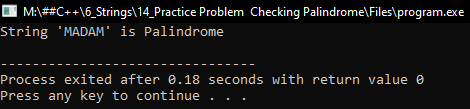# How to check if a string is Palindrome or not in C++

## How to check if a string is Palindrome or not in C++

In this article, I am going to discuss How to check if a string is Palindrome or not in C++ Language with examples. Please read our previous article, where we discussed How to Count Vowels, Consonants, and Words in a String in C++ with examples.

##### How to check if a string is Palindrome or not in C++?

Here, we will write a program that will check if a string is palindrome or not. Palindrome means the string remains the same after reversing the characters. For example – ‘MADAM’, ‘NAMAN’. These are palindromes as they remain the same after reversing the order of letters. Now let us see the program for this.

##### Program to Check a Given string is Palindrome or not:

Given string str consisting of N number of characters of the English alphabet. We need to write a program to check if the given string is a palindrome or not using the C++ language. If the given string is a palindrome, then print “Palindrome“. Otherwise, print “Not a Palindrome“. A string is said to be palindrome if the reverse of the string is the same as the string. The below example code exactly does the same thing.

```#include <iostream>
#include <string.h>
using namespace std;
int main()
{
string rev = "";
int len = (int) str.length ();
rev.resize (len);

for (int i = 0, j = len - 1; i < len; i++, j--)
{
rev[i] = str[j];
}
rev[len] = '\0';

cout << "String '" << str << "' is ";

if (str.compare (rev) == 0)
cout << "Palindrome" << endl;
else
cout << "Not a Palindrome" << endl;

return 0;
}
```
##### Output:##### Using STL Library Function to Check whether a string is a Palindrome or Not in C++ Language:

We can also use the inbuilt reverse function which is available in STL to check whether a string is a palindrome or not. Please follow the below steps to solve the problem:

1. First, copy the string Str to another string, say str2, and then reverse the string Str.
2. Now check if the string Str is equal to the string str2 and then print Palindrome. Otherwise, print Not a Palindrome.

The following code is the implementation of the above approach.

```#include <bits/stdc++.h>
using namespace std;

// Function to check whether the string is palindrome or not
string IsPalindrome (string Str)
{
// Stores the reverse of the string Str
string Str2 = Str;

// Reverse the string Str
reverse (Str.begin (), Str.end ());

// If Str is equal to Str2
if (Str == Str2)
{
// Return "Palindrome"
return "Palindrome";
}
// Otherwise
else
{
// return "Not a Palindrome"
return "Not a Palindrome";
}
}

int main()
{
string S = "NAMAN";
cout << IsPalindrome (S);
return 0;
}
```

Output: Palindrome

##### Efficient Approach:

The above approach can be optimized in terms of space complexity by traversing the string and checking whether the character at ith index is equal to the character at the (N-i-1)th index for every index in the range [0, N/2]. Please follow the below steps to solve the problem:

1. Iterate over the range [0, N/2], using the variable i and in each iteration check if the character at index i and N-i-1 are not equal, then print Not a Palindrome and break.
2. If none of the above cases satisfy, then print Palindrome.

The following code is the implementation of the above approach:

```#include <bits/stdc++.h>
using namespace std;

// Function to check whether the string is palindrome or not
string IsPalindrome (string Str)
{
// Iterate over the Range [0, N/2]
for (int i = 0; i < Str.length () / 2; i++)
{
// If Str[i] is not equal to the Str[N-i-1]
if (Str[i] != Str[Str.length () - i - 1])
{
// Return Not a Palindrome
return "Not a Palindrome";
}
}
// Return "Palindrome"
return "Palindrome";
}

int main()
{
string S = "NAMAN";
cout << IsPalindrome (S);
return 0;
}
```

Output: Palindrome

Time Complexity: O(N)
Auxiliary Space: O(1)